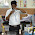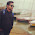# SPINELS (Normal or Inverse)

Spinel is a name of Mixed Ore of Magnesium(Mg) and Aluminium(Al), its molecular formula is MgAlO4 (MgO.Al2O3). Here Mg is in +2 oxid. state and Al is in +3 oxid. state.
So, in general we can say Spinels are AB2X4 type complexes
where, A= any dipositive metal (eg. Mg+2, Ni+2
B= any tripositive metal (eg. Fe+3, Al+3, La+3)
X = O, S, Se etc.

### General Properties Of Spinels

1. Spinels are mixed oxides having formula AO.B2O3
2. They have Face Centred Cubic (CCP) structures.
3. Total No. of Atoms per unit cell = 4
• No. of Tetrahedral (Td) voids per unit cell = 8
• No. of Octahedral (Oh) voids per unit cell = 4

### TYPES OF SPINELS:

On the basis of position occupied by A+2 ions and  B+3 ions, spinels are of two types:

#### Normal Spinel:

The divalent A+2 ions occupy the tetrahedral voids, whereas the trivalent B+3 ions occupy the octahedral voids in the close packed arrangement of oxide ions.  A normal spinel can be represented as: (A+2)tet(B+3)2octO4
e.g. Mn3O4, ZnFe2O4, FeCr2O4

#### Inverse Spinel:

The A+2 ions occupy the octahedral voids, whereas half of B+3 ions occupy the tetrahedral voids.
It can be represented as: (B+3)tet(A+2B+3)octO4  E.g. Fe3O4 (ferrite), CoFe2O4, NiFe2O4 etc.

### Prediction Of Structure Of Spinels:

basic rule for determining whether a complex is Normal or Inverse Spinel

• For Normal Spinel:
CFSE of B+3 ions in Octahedral Feild > CFSE of B+3 ions in Tetrahedral Feild
>CFSE of A+2 ions in Octahedral Feild
>CFSE of A+2 ions in Tetrahedral Feild
[i.e. if CFSE of B+3 ions in Octahedral Feild is largest of all CFSE]

• For Inverse Spinel:
CFSE of A+2  ions in Octahedral Feild > CFSE of A+2 ions in Tetrahedral Feild
>CFSE of B+3 ions in Octahedral Feild
>CFSE of B+3 ions in Tetrahedral Feild

[i.e. if CFSE of A+2 ions in Octahedral Feild is largest of all CFSE]

Let's take an example of Mn3O4
Here Mn3Oexist as Mn.Mn2O4, therefore Mn exists in +2 and +3 oxidation state.

CFSE of Mn+2 in
octahedral feild = 0o

CFSE of Mn+2 in
tetrahedral feild = 0t
CFSE of Mn+3 in
octahedral feild = -0.6∆0
CFSE of Mn+3 in
tetrahedral feild = -0.4∆t

here CFSE of Mn+3 in Oh feild is more than all, therefore is Mn3O4 Normal Spinel

#### Rules:

1. If both  A+2 and  B+3 are non transition metals then spinel will be NORMAL.
2. If A+2  is Non transition metal and B+3 is transition metal (having d1 d2 d3 d4 d6 d7 d8 d9) then spinel will be NORMAL.
3. If is transition metal with (d1 d2 d3 d4 d6 d7 d8 d9 ) and B is  also transition metal with (d0 d5 and d10) system then spinel will be INVERSE.

### EXAMPLES FOR SPINEL AND INVERSE SPINEL STRUCTURES

1) MgAl2O4 is a normal spinel since both the divalent and trivalent ions are non transition metal ions. There is no question of CFSE.

2) Mn3O4 is a normal spinel since the Mn2+ ion is a high spin d5 system with zero LFSE. Whereas, Mn3+ ion is a high spin d4 system with considerable LFSE.

3) Fe3O4 is an inverse spinel since the Fe(III) ion is a high spin d5 system with zero CFSE. Whereas the divalent Fe(II) is a high spin d6 system with more CFSE.

4) NiFe2O4 is again an inverse spinel since the divalent Ni2+ (a d8 ion) has more CFSE than the trivalent Fe3+ (a d5 ion).

5) FeCr2O4 is a normal spinel since the divalent Fe2+ is a high spin d6 ion with CFSE = 4 Dq and the trivalent Cr3+ is a high spin d3 ion with CFSE = 12 Dq. Hence Cr3+ gets more OSSE while occupying octahedral sites.

6) Co3O4 is a normal spinel. Even in the presence of weak field oxo ligands, the Co3+ is a low spin d6 ion with very high CFSE. It is due to high charge on Co3+. Hence all the Co3+ ions occupy the octahedral sites.

### Exception:

• NiAl2O4 for which the formula can be written as (Al0.75Ni0.25)tet [Ni0.75Al1.25]octaO is a Random Defected Inverse Spinel. The CFSE of NiII is greater in octahedral than tetrahedral coordination. But Al3+ also has strong preference for octahedral sites due to high lattice energy. This leads to almost complete randomization of all the cations on all the available sites. Its formula can be written as (Al0.75Ni0.25)tet [Ni0.75Al1.25]octaO4.

•  FeAl2O4 is Normal Spinel because  has stronger preference for Oh sites due to its High Lattice Energy

Like Our Facebook Page and stay connected with us:

***Did You Liked The Post, Do Tell Us In Comment Section Below***#### About Noorul Huda -

A chemist, a teacher and a passionate blogger. Currently pursuing his PhD from School of Chemistry, University of Hyderabad is creative head of this blog and lives with a motto of teaching what he knows and exploring what he don't.

Subscribe to this Website via Email :Noorul Huda
AUTHOR